IdentifiantMot de passe
Mot de passe oublié ?

Inscrivez-vous gratuitement
pour pouvoir participer, suivre les réponses en temps réel, voter pour les messages, poser vos propres questions et recevoir la newsletter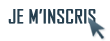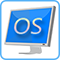Discussion :

# Condition qui ne fonctionne pas!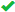Sujet :

## Cpcdos

1.Condition qui ne fonctionne pas!
Salut à tous les Cpdosiens!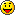Ah, ça faisais longtemps que je n'avais pas remis les pieds dans CPCDOS.
Il y a 2 semaines de ça, j'ai repris la programmation à 0 de HOS et je compte bien cette fois-ci finir la version 1.0.
Malheureusement je viens de tomber sur une impasse et je n'arrive pas à m'en dépêtrer.
Voilà, je suis rendus dans la programmation des paramètres de HOS, plus particulièrement les paramètres utilisateur (Mot de passe et nom de compte).
Pour changer de mot de passe j'ai mis une sécurité de vérification (Tapez 2 fois le MDP).
A ce moment là de mon code j'ai mis un bouton enregistrer qui dans son EV récupère le contenus des deux MDP rentré et du compte utilisateur rentré.
Ensuite il vérifie si MDP1 = MDP2, sauf que c'est tout le temps vrais même si le mot de passe n'est pas le même (j'ai vérifier à partir de message box).
Je suis sur que c'est une erreur très bête mais je ne la trouve pas...Je vous met le code (La condition avec le bouton enregistrer est à la fin du code). Bonne chance, il y a 183 ligne!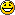Code : Sélectionner tout - Visualiser dans une fenêtre à part
```123456789101112131415161718192021222324252627282930313233343536373839404142434445464748495051525354555657585960616263646566676869707172737475767778798081828384858687888990919293949596979899100101102103104105106107108109110111112113114115116117118119120121122123124125126127128129130131132133134135136137138139140141142143144145146147148149150151152153154155156157158159160161162163164165166167168169170171172173174175176177178179180181182183REM/ Section Utilisateur de Parametres

:INFO:
REM/ Informations Personnels de l'Utilisateur
EXE/ & %SYSTEME%\PARA\EFF.CPC

FIX/ MDP1 = %MDPV%
FIX/ MDP2 = %MDPV%
FIX/ COMPTE1 = %COMPTEV%

INI/ LABEL(
INI;FENETRE = "PARA"
INI;NOM = "IFOP_LBL2"
INI;TEXTE = "Informations"
INI;COULEURF = "%COULFD_PARA%"
INI;COULEURP = "255,255,255"
INI;TRANSPARENT = "0"
INI;TYPE = "0"
INI;TX = "0"
INI;TY = "0"
INI;PX = "199"
INI;PY = "10"
CREER/
INI/ LABEL)

INI/ IMAGEBOX(
INI;NOM = "RETOUR"
INI;FENETRE = "PARA"
INI;COULEUR = "000,000,000"
INI;COULEURF = "001,001,001"
INI;TYPE = "0"
INI;IMAGE = "%MEDIA%\ICONES\PREC.BMP"
INI;PX = "10"
INI;PY = "10"
INI;TX = "13"
INI;TY = "13"
CREER/
EV/ %SYSTEME%\PARA\EFF.CPC
INI/ IMAGEBOX)

INI/ IMAGEBOX(
INI;NOM = "IMG_UTL"
INI;FENETRE = "PARA"
INI;COULEUR = "000,000,000"
INI;COULEURF = "001,001,001"
INI;TYPE = "0"
INI;IMAGE = "%MEDIA%\IUG\PERSOB.BMP"
INI;PX = "430"
INI;PY = "18"
INI;TX = "50"
INI;TY = "50"
CREER/
INI/ IMAGEBOX)

INI/ TEXTEBOX(
INI;NOM = "NUTL"
INI;FENETRE = "PARA"
INI;TYPE = "1"
INI;COULEURF = "255,255,255"
INI;COULEURP = "000,000,000"
INI;TEXTE = "%COMPTEV%"
INI;PX = "225"
INI;PY = "45"
INI;TX = "175"
INI;TY = "15"
CREER/
INI/ TEXTEBOX)

INI/ LABEL(
INI;FENETRE = "PARA"
INI;NOM = "NUTL_LBL"
INI;TEXTE = "Nom du compte:"
INI;COULEURF = "%COULFD_PARA%"
INI;COULEURP = "255,255,255"
INI;TRANSPARENT = "0"
INI;TYPE = "0"
INI;TX = "0"
INI;TY = "0"
INI;PX = "10"
INI;PY = "52"
CREER/
INI/ LABEL)

INI/ TEXTEBOX(
INI;NOM = "CMDP"
INI;FENETRE = "PARA"
INI;TYPE = "P1"
INI;COULEURF = "255,255,255"
INI;COULEURP = "000,000,000"
INI;TEXTE = "%MDPV%"
INI;PX = "225"
INI;PY = "65"
INI;TX = "175"
INI;TY = "15"
CREER/
INI/ TEXTEBOX)

INI/ TEXTEBOX(
INI;NOM = "CMDP2"
INI;FENETRE = "PARA"
INI;TYPE = "P1"
INI;COULEURF = "255,255,255"
INI;COULEURP = "000,000,000"
INI;TEXTE = "%MDPV%"
INI;PX = "225"
INI;PY = "85"
INI;TX = "175"
INI;TY = "15"
CREER/
INI/ TEXTEBOX)

INI/ LABEL(
INI;FENETRE = "PARA"
INI;NOM = "CMDP_LBL"
INI;TEXTE = "Mot de passe du compte:"
INI;COULEURF = "%COULFD_PARA%"
INI;COULEURP = "255,255,255"
INI;TRANSPARENT = "0"
INI;TYPE = "0"
INI;TX = "0"
INI;TY = "0"
INI;PX = "10"
INI;PY = "72"
CREER/
INI/ LABEL)

INI/ LABEL(
INI;FENETRE = "PARA"
INI;NOM = "CMDP_LBL2"
INI;TEXTE = "Verification:"
INI;COULEURF = "%COULFD_PARA%"
INI;COULEURP = "255,255,255"
INI;TRANSPARENT = "0"
INI;TYPE = "0"
INI;TX = "0"
INI;TY = "0"
INI;PX = "10"
INI;PY = "92"
CREER/
INI/ LABEL)

INI/ IMAGEBOX(
INI;NOM = "ENRG"
INI;FENETRE = "PARA"
INI;COULEUR = "000,000,000"
INI;COULEURF = "001,001,001"
INI;TYPE = "0"
INI;IMAGE = "%MEDIA%\IUG\ENRG.BMP"
INI;PX = "370"
INI;PY = "285"
INI;TX = "100"
INI;TY = "30"
CREER/
EV/ %SYSTEME%\PARA\UTL.CPC
INI/ IMAGEBOX)

PROC/ ENRG(CLIC)
FIX/ MDP1 = %MDPV%
FIX/ MDP2 = %MDPV%

INI/ TEXTEBOX(
INI; NOM = "NUTL"
INI; TEXTE = "#%COMPTE1"
INI/ TEXTEBOX)

INI/ TEXTEBOX(
INI; NOM = "CMDP"
INI; TEXTE = "#%MDP1"
INI/ TEXTEBOX)

INI/ TEXTEBOX(
INI; NOM = "CMDP2"
INI; TEXTE = "#%MDP2"
INI/ TEXTEBOX)

SI/ %MDP1% N %MDP2% (:
EXE/ %SYSTEME%\PARA\ERREUR.CPC /l:MDP
STOP/
FIN/ SI

EXE/ %SYSTEME%\PARA\ENR.CPC /l:INFO_UTL
STOP/
FIN/ PROC```
PS: Sébastien si tu vois ce message, il est impossible d'accéder aux paramètres utilisateur de CraftyOS car il n'y a pas de procédure qui a été faite dans le fichier CFG_EV.CPC!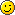Répondre avec citation   0  0

2.Salut,

Essaye ça:
 Code : Sélectionner tout - Visualiser dans une fenêtre à part
```1234SI/ %MDP1% = %MDP2 (:
EXE/ %SYSTEME%\PARA\ERREUR.CPC /l:MDP
STOP/
FIN/ SI```
Si ça marche toujours pas, redemandeMINEOSX, Le créateur de MINEOSX et de MineShell 1.0Répondre avec citation   1  0

3.Re et merci de ta réponse,
Malheureusement cela ne marche pas.
Peut-tu m'expliquer pour quoi sur les ligne 2, 3 et 4 de la condition tu as mis une tabulation supplémentaire?Répondre avec citation   0  0

4.Re
C'est pour faire jolie à la conditionJe sais pas te quoi dire c'est peut être une erreur que t'as faite au début du code, reverifie tout ce que tu as fait.

MINEOSX, Le Créateur de MINEOSX et de MineShell 1.0.Répondre avec citation   0  0

5.Merci de ton aide!Répondre avec citation   0  0

6.Salut! J'ai copié ton code je l'ai testé, j'ai cherché cherché, j'arrivais pas à savoir d'où venais le bug, j'ai finalement trouvé, alors premièrement promet nous de tuer personne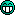.

Le problème est situé à l'endroit où tu récupère les propriétés
 Code : Sélectionner tout - Visualiser dans une fenêtre à part
```12345678910111213    INI/ TEXTEBOX(
INI; NOM = "NUTL"
INI; TEXTE = "#%COMPTE1"
INI/ TEXTEBOX)
INI/ TEXTEBOX(
INI; NOM = "CMDP1"
INI; TEXTE = "#%MDP1"
INI/ TEXTEBOX)

INI/ TEXTEBOX(
INI; NOM = "CMDP2"
INI; TEXTE = "#%MDP2"
INI/ TEXTEBOX)```
Entre INI; et le nom de propriété tu as mis un espace " ". Oui c'est très bête, mais c'est aussi de ma faute que Cpcdos ne mette pas une alerte..

Voici la correction à copier :
 Code : Sélectionner tout - Visualiser dans une fenêtre à part
```12345678910111213    INI/ TEXTEBOX(
INI;NOM = "NUTL"
INI;TEXTE = "#%COMPTE1"
INI/ TEXTEBOX)
INI/ TEXTEBOX(
INI;NOM = "CMDP1"
INI;TEXTE = "#%MDP1"
INI/ TEXTEBOX)

INI/ TEXTEBOX(
INI;NOM = "CMDP2"
INI;TEXTE = "#%MDP2"
INI/ TEXTEBOX)```
CordialementRépondre avec citation   1  0

7.Salut et merci Seb!
Je ne sais pas si tu as vu la fin de mon premier message mais il est impossible d'accéder aux paramètres utilisateur de CraftyOS car il n'y a pas de procédure qui a été faite dans le fichier CFG_EV.CPC!Répondre avec citation   0  0

8.Re, j'ai tester mais cela ne marche pas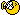, je suis presser donc je fais ce message vite fait!

Voilà le code de la procédure modifiée:
 Code : Sélectionner tout - Visualiser dans une fenêtre à part
```123456789101112131415161718192021222324PROC/ ENRG(CLIC)
INI/ TEXTEBOX(
INI;NOM = "NUTL"
INI;TEXTE = "#%COMPTE1"
INI/ TEXTEBOX)

INI/ TEXTEBOX(
INI;NOM = "CMDP"
INI;TEXTE = "#%MDPPP"
INI/ TEXTEBOX)

INI/ TEXTEBOX(
INI;NOM = "CMDP2"
INI;TEXTE = "#%MDPP"
INI/ TEXTEBOX)

SI/ %MDP1% = %MDP2% (:
EXE/ %SYSTEME%\PARA\ENR.CPC /l:INFO_UTL
STOP/
SINON/
EXE/ %SYSTEME%\PARA\ERREUR.CPC /l:MDP
STOP/
FIN/ SI
FIN/ PROC```Répondre avec citation   0  0

9.Salut tout le monde!C'est bon j'ai réglé mon problème, je vous laisse le code pour ceux que ça intéresse:
 Code : Sélectionner tout - Visualiser dans une fenêtre à part
```123456789101112131415161718192021222324252627282930313233343536373839404142434445464748495051525354555657585960616263646566676869707172737475767778798081828384858687888990919293949596979899100101102103104105106107108109110111112113114115116117118119120121122123124125126127128129130131132133134135136137138139140141142143144145146147148149150151152153154155156157158159160161162163164165166167168169170171172173174175176177178179180181182183REM/ Section Utilisateur de Parametres

:INFO:
REM/ Informations Personnels de l'Utilisateur
EXE/ & %SYSTEME%\PARA\EFF.CPC

FIX/ MDP1 = %MDPV%
FIX/ MDP2 = %MDPV%
FIX/ COMPTE1 = %COMPTEV%

INI/ LABEL(
INI;FENETRE = "PARA"
INI;NOM = "IFOP_LBL2"
INI;TEXTE = "Informations"
INI;COULEURF = "%COULFD_PARA%"
INI;COULEURP = "255,255,255"
INI;TRANSPARENT = "0"
INI;TYPE = "0"
INI;TX = "0"
INI;TY = "0"
INI;PX = "199"
INI;PY = "10"
CREER/
INI/ LABEL)

INI/ IMAGEBOX(
INI;NOM = "RETOUR"
INI;FENETRE = "PARA"
INI;COULEUR = "000,000,000"
INI;COULEURF = "001,001,001"
INI;TYPE = "0"
INI;IMAGE = "%MEDIA%\ICONES\PREC.BMP"
INI;PX = "10"
INI;PY = "10"
INI;TX = "13"
INI;TY = "13"
CREER/
EV/ %SYSTEME%\PARA\EFF.CPC
INI/ IMAGEBOX)

INI/ IMAGEBOX(
INI;NOM = "IMG_UTL"
INI;FENETRE = "PARA"
INI;COULEUR = "000,000,000"
INI;COULEURF = "001,001,001"
INI;TYPE = "0"
INI;IMAGE = "%MEDIA%\IUG\PERSOB.BMP"
INI;PX = "430"
INI;PY = "18"
INI;TX = "50"
INI;TY = "50"
CREER/
INI/ IMAGEBOX)

INI/ TEXTEBOX(
INI;NOM = "NUTL"
INI;FENETRE = "PARA"
INI;TYPE = "1"
INI;COULEURF = "255,255,255"
INI;COULEURP = "000,000,000"
INI;TEXTE = "%COMPTEV%"
INI;PX = "225"
INI;PY = "45"
INI;TX = "175"
INI;TY = "15"
CREER/
INI/ TEXTEBOX)

INI/ LABEL(
INI;FENETRE = "PARA"
INI;NOM = "NUTL_LBL"
INI;TEXTE = "Nom du compte:"
INI;COULEURF = "%COULFD_PARA%"
INI;COULEURP = "255,255,255"
INI;TRANSPARENT = "0"
INI;TYPE = "0"
INI;TX = "0"
INI;TY = "0"
INI;PX = "10"
INI;PY = "52"
CREER/
INI/ LABEL)

INI/ TEXTEBOX(
INI;NOM = "CMDP"
INI;FENETRE = "PARA"
INI;TYPE = "P1"
INI;COULEURF = "255,255,255"
INI;COULEURP = "000,000,000"
INI;TEXTE = "%MDPV%"
INI;PX = "225"
INI;PY = "65"
INI;TX = "175"
INI;TY = "15"
CREER/
INI/ TEXTEBOX)

INI/ TEXTEBOX(
INI;NOM = "CMDP2"
INI;FENETRE = "PARA"
INI;TYPE = "P1"
INI;COULEURF = "255,255,255"
INI;COULEURP = "000,000,000"
INI;TEXTE = "%MDPV%"
INI;PX = "225"
INI;PY = "85"
INI;TX = "175"
INI;TY = "15"
CREER/
INI/ TEXTEBOX)

INI/ LABEL(
INI;FENETRE = "PARA"
INI;NOM = "CMDP_LBL"
INI;TEXTE = "Mot de passe du compte:"
INI;COULEURF = "%COULFD_PARA%"
INI;COULEURP = "255,255,255"
INI;TRANSPARENT = "0"
INI;TYPE = "0"
INI;TX = "0"
INI;TY = "0"
INI;PX = "10"
INI;PY = "72"
CREER/
INI/ LABEL)

INI/ LABEL(
INI;FENETRE = "PARA"
INI;NOM = "CMDP_LBL2"
INI;TEXTE = "Verification:"
INI;COULEURF = "%COULFD_PARA%"
INI;COULEURP = "255,255,255"
INI;TRANSPARENT = "0"
INI;TYPE = "0"
INI;TX = "0"
INI;TY = "0"
INI;PX = "10"
INI;PY = "92"
CREER/
INI/ LABEL)

INI/ IMAGEBOX(
INI;NOM = "ENRG"
INI;FENETRE = "PARA"
INI;COULEUR = "000,000,000"
INI;COULEURF = "001,001,001"
INI;TYPE = "0"
INI;IMAGE = "%MEDIA%\IUG\ENRG.BMP"
INI;PX = "370"
INI;PY = "285"
INI;TX = "100"
INI;TY = "30"
CREER/
EV/ %SYSTEME%\PARA\UTL.CPC
INI/ IMAGEBOX)

PROC/ ENRG(CLIC)
FIX/ MDP1 = %MDPV%
FIX/ MDP2 = %MDPV%

INI/ TEXTEBOX(
INI;NOM = "NUTL"
INI;TEXTE = "#%COMPTE1"
INI/ TEXTEBOX)

INI/ TEXTEBOX(
INI;NOM = "CMDP"
INI;TEXTE = "#%MDP1"
INI/ TEXTEBOX)

INI/ TEXTEBOX(
INI;NOM = "CMDP2"
INI;TEXTE = "#%MDP2"
INI/ TEXTEBOX)

SI/ %MDP1% N %MDP2% (:
EXE/ %SYSTEME%\PARA\ERREUR.CPC /l:MDP
STOP/
FIN/ SI

EXE/ %SYSTEME%\PARA\ENR.CPC /l:INFO_UTL
STOP/
FIN/ PROC```Répondre avec citation   0  0

+ Répondre à la discussion
Cette discussion est résolue.
 Actualités COURS SYSTEMES LIVRES SYSTEMES UNIX BSD